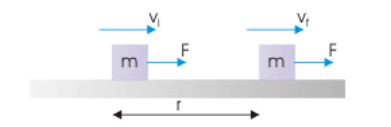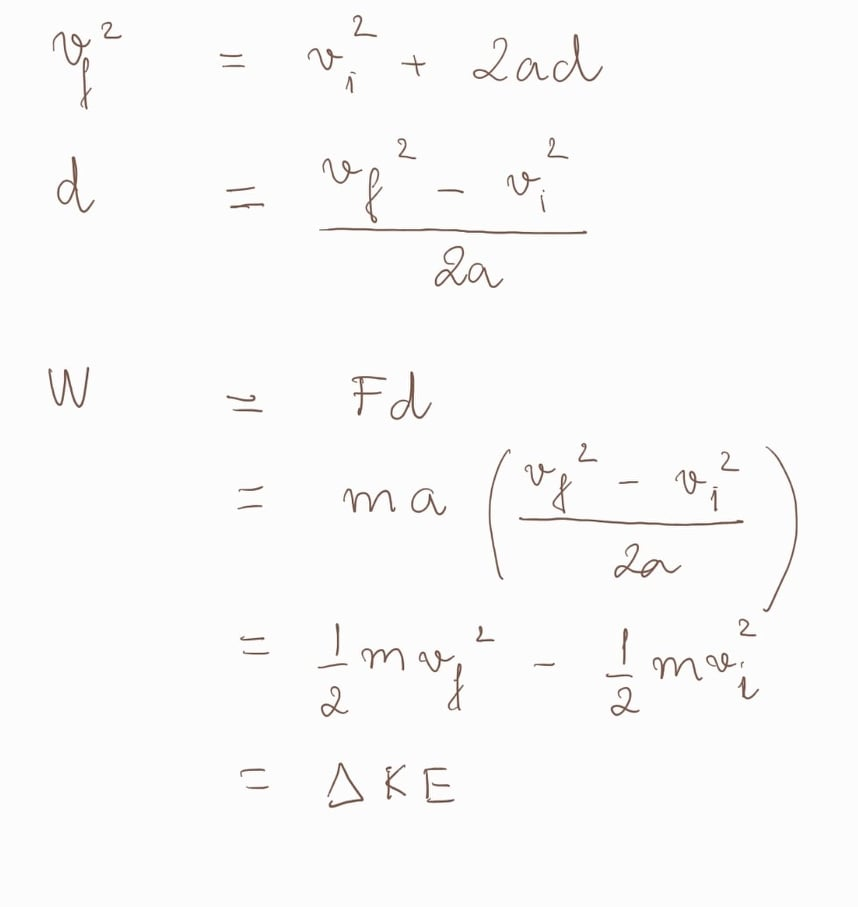Cyber Sale - Only 3 Days Remaining: ALL MCAT Products & Admissions Services

MCAT Content / Work / Work Kinetic Energy Theorem

### Work Kinetic Energy Theorem

Topic: Work

The work-energy theorem states that the work done by all forces acting on a particle equals the change in the particle’s kinetic energy.

A force does work on the block and sets it in motion. The kinetic energy of the block (the energy that it possesses due to its motion) increases as a result of the amount of work.Vi and Vf is the velocity of the particle before and after the application of force, and m is the particle’s mass.With the knowledge of this relationship, try the energy approach first before applying kinematics when solving a problem, as the energy approach is much easier.

Practice Questions

MCAT Official Prep (AAMC)

Sample Test C/P Section Question 57

Practice Exam 1 C/P Section Passage 4 Question 21

Practice Exam 3 C/P Section Passage 2 Question 7

Key Points

• The work W done by the net force on a particle equals the change in the particle’s kinetic energy KE

• The work-energy theorem can be derived from Newton’s second law.

• Prioritize energy approach to kinematics in problem-solving.

Key Terms

the kinetic energy of an object is the energy that it possesses due to its motion

work: a measure of energy transfer that occurs when an object is moved over a distance by an external force

Billing Information